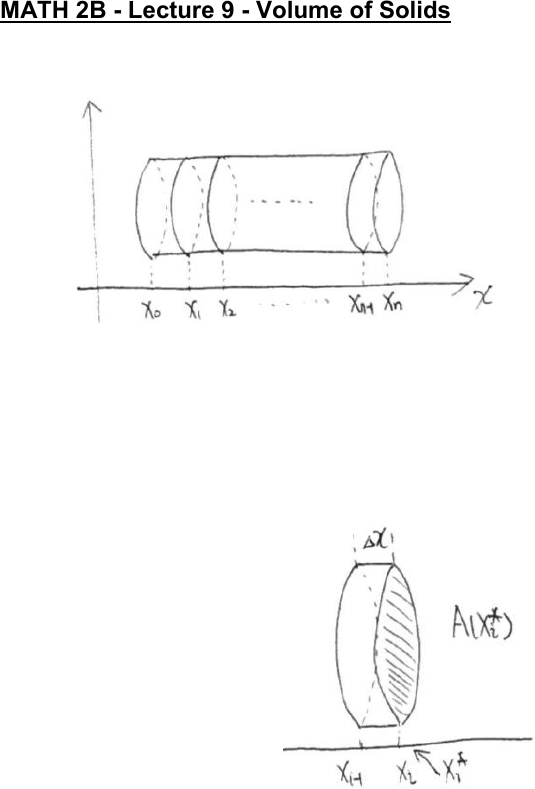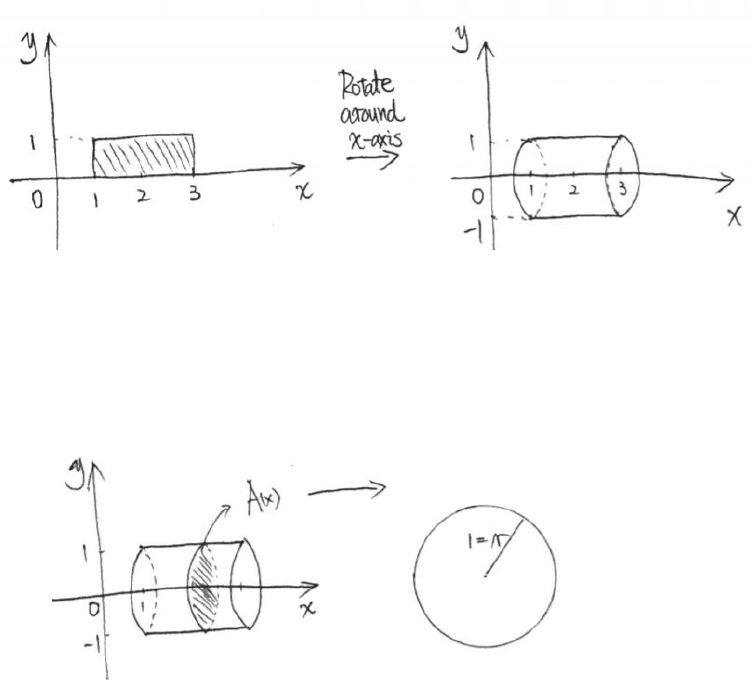Class Notes (1,100,000)
US (460,000)
UC-Irvine (10,000)
MATH (1,000)
MATH 2B (600)
Lecture 9

# MATH 2B Lecture Notes - Lecture 9: Riemann SumPremium

Department
Mathematics
Course Code
MATH 2B
Professor
ERJAEE, G.
Lecture
9

This preview shows page 1. to view the full 5 pages of the document.01/25/2019
MATH 2B - Lecture 9 - Volume of Solids
Consider a general cylinder:
To calculate the volume of this general solid, we cut it into many slices vertically.
In general, for the ith slice, we use a short cylinder to approximate its volume.
 
Again, we assume each slice has the same height We use the area at the
sample point as the base.
 
base height
Then we add them together to obtain the
approximation for the whole solid.
 

Then we take the limit of the above Riemann Sum:
 



A(x): the area of intersecting face in term of x
Example:

Unlock to view full version

Subscribers Only

Only page 1 are available for preview. Some parts have been intentionally blurred.

Subscribers OnlyFind the volume obtained by rotating the region bounded by y=1, on [1,3] around
x-axis.
Solution:
By rotating the rectangular region, we obtain a cylinder.
Now we try to write down the definite integral for its volume:
1. Treat x as variable (since it’s rotating around the x-axis)
2. Cut the solid vertically, and find the area of intersecting plane A(x) as a
function of x.
Cutting area is a circle with a constant radius r=1 → A(x) = πr² = π
3. Set up the integral
 
 
  
Example:
Compute the volume obtained by rotating the region s with x-axis which is
bounded by f(x)=x² on [0,1].